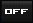View Single Post01-23-2010, 07:57 AM #884 Initiate Avalon Senior Member   Join Date: Aug 2009 Location: New Zealand Posts: 391Re: Thuban Q&A: (warning longer than normal posts here) [QUOTE=abraxasinas;227115]The 'big picture' encompasses your cosmic ID of being within Spacetime simultaneously with being without it. Initiate asked a very good question. How can something exist in nothing? Knowing a little mathematics, envisage an expanding series of the form: 0/1+1/2+2/3+3/4+...+n/(n+1)+...99999/1000000+...always getting bigger as a fraction or a decimal but never actually reaching the limit of 1/1=1. This way, the Mirror of the Infinity in 0/0=∞/∞=1, because 0=0 and ∞=∞ to define the inversion of 1/1=1 in the undefinability of 1/0≡∞ ↔ ∞.0≡1 and limited in the mathematical symbolisation in the physicalisations of limits in 1/∞→0 and 1/0→∞ as the reciprocities of each other by 0/1≡0 and ∞/1≡∞. All of Creation = 1 The Void = 0 The Eternity = 1/0=Infinity meaning that 0xInfinity=1! (not in the physicalised limit but by definition) if we take the algebra a / b = c then c * b = a. I understand this to be a fundemental law of mathematics. Now, in order for us to calculate c, when a = 1 and b = ∞ then 1/∞ = c and c * ∞ = 1 what is c? c can not equal 0 because if 1/∞ = 0 (c) then 0 (c) * ∞ = 0 and not 1 c must be a number infinitely close to 0 but not equal to 0. so 0 * ∞ doesn't equal 1 The Eternity = 1/0=Infinity meaning that 0xInfinity=1 is false near 0 (c) * ∞ can = 1 but c can not be defined on its own. am I missing something?